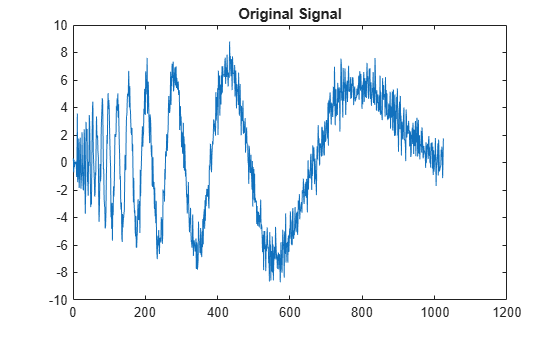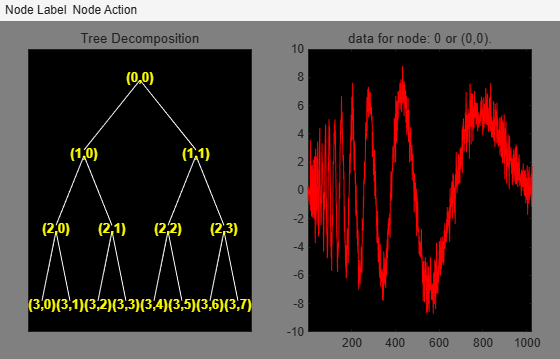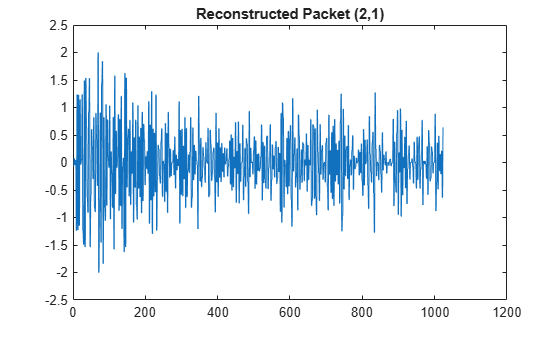# wprcoef

Reconstruct wavelet packet coefficients

## Syntax

``X = wprcoef(T)``
``X = wprcoef(T,N)``

## Description

````X = wprcoef(T)` reconstructs coefficients of the node `0` of the wavelet packet tree `T`. `wprcoef` is a one- or two-dimensional wavelet packet analysis function. ```

example

````X = wprcoef(T,N)` reconstructs coefficients of the node `N` of the wavelet packet tree `T`.```

## Examples

collapse all

Load and plot original signal. The function uses zero padding as an extension mode for dealing with the problem of border distortion in signal or image analysis. For more information, see `dwtmode`.

```load noisdopp; s = noisdopp; plot(s); title('Original signal');```Decompose the original signals at depth 3 with `db1` wavelet packets using Shannon entropy.

`T = wpdec(s,3,'db1','shannon');`

Plot the wavelet packet tree.

`plot(T)`Reconstruct the packet at node (2,1).

`X = wprcoef(T,[2 1]);`

Plot the reconstructed packet.

```plot(X); title('Reconstructed packet (2,1)');```## Input Arguments

collapse all

Wavelet packet tree, specified as a `wptree` object.

Node in the wavelet packet tree T, specified as a nonnegative integer or as a pair of nonnegative integers.

## Output Arguments

collapse all

Reconstructed coefficients of the wavelet packet, returned as a row vector.

## Version History

Introduced before R2006a